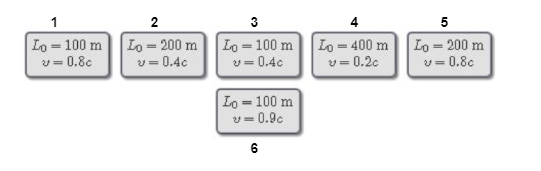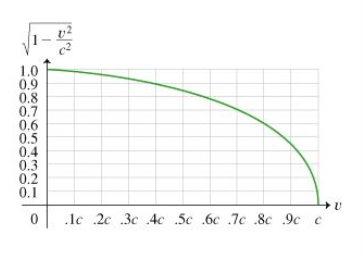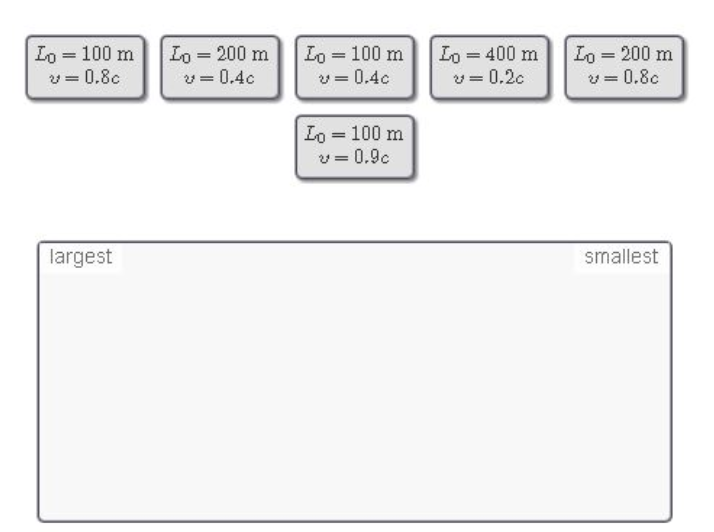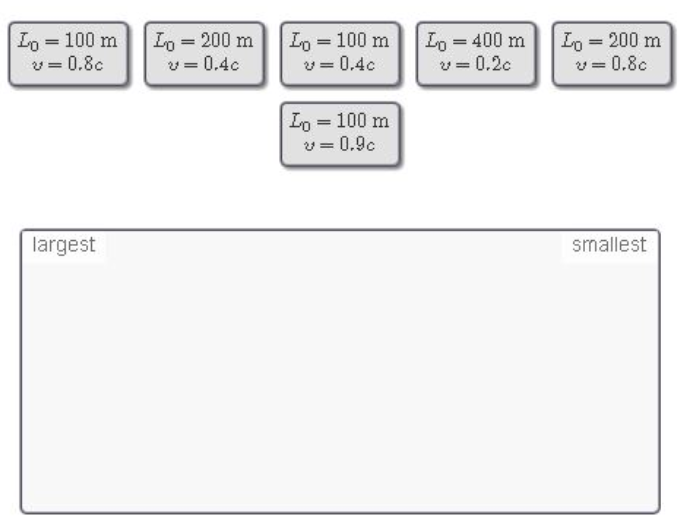# Problem: Six spaceships with rest lights L0 zoom past an intergalactic speed trap. The officer on duty records the speed of each ship , v. (No ship is going in excess of the stated speed limit of c, so she doesn't have to pull anyone over for a ticker.) Part A. Rank these spaceships on the basis of their length measured by the police officer. Part BRank these spaceships on the basis of their lengths as measured by their respective captains.

###### FREE Expert Solution

Part A

The officer sees the contracted length of the spaceship.

This length is given by:

$\overline{){\mathbf{L}}{\mathbf{=}}{{\mathbf{L}}}_{{\mathbf{0}}}\sqrt{\mathbf{1}\mathbf{-}\frac{{\mathbf{v}}^{\mathbf{2}}}{{\mathbf{c}}^{\mathbf{2}}}}}$

From the graph we can use:

$\mathbit{L}\mathbf{=}\sqrt{\mathbf{1}\mathbf{-}\frac{{\mathbf{v}}^{\mathbf{2}}}{{\mathbf{c}}^{\mathbf{2}}}}$, for a particular v.

Label the spaceships as follows:(1) L = (100)(0.6) = 60 m

(2) L = (200)(0.9) = 180 m

(3) L = (100)(0.9) = 90 m

79% (331 ratings)###### Problem Details

Six spaceships with rest lights L0 zoom past an intergalactic speed trap. The officer on duty records the speed of each ship , v. (No ship is going in excess of the stated speed limit of c, so she doesn't have to pull anyone over for a ticker.)Part A.

Rank these spaceships on the basis of their length measured by the police officer.Part B

Rank these spaceships on the basis of their lengths as measured by their respective captains.